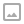## Simple Force Directed Graph in Unity

·   ·  ☕ 2 min read

Lets riff on how to draw force directed graphs in Unity. A force directed graph is a directed graph that is drawn and positioned by having each node apply force to every other node. Nodes will repel other disconnected nodes and attract connected nodes.

I have only a basic understanding of how these work and have been trying to read up on how to implement them better. To learn a bit more we’ll be implementing a basic directed graph in C# and then using Unity’s Gizmo’s to quickly render out the result. This is quick and basic but gets us to the point we need.

For applying forces we’ll use logarithms for attraction and exponential functions for repelling other nodes. Determining the disconnected nodes is fairly easy (though not that efficient) and can be done by taking all nodes except the children of the target node. This is simple and can be done in a single line using LINQ. Once we have that it’s just a basic pair of loops to apply the two forces to each node.

Clearly this algorithm has some issues, especially with speed, but it has the advantage of being relatively simple to build. Now that we have it working in a simple fashion we can start refining and improving its efficiency.

Here is an example of a Force Directed Graph rendered with D3 a Javascript framework. It demonstrates the final goal we’re going for (though we are moving from 2D to 3D): https://bl.ocks.org/mbostock/4062045

Wikipedia has a brief description and example on what Force Directed Graphs are and how to draw them: en.wikipedia.org/wiki/Force-directed_graph_drawing

This paper from Brown University walks through some of the math behind Force Directed Graphs, it’s where the formula we use in the video come from: https://cs.brown.edu/~rt/gdhandbook/chapters/force-directed.pdf

Discord: https://discord.gg/hU5Kq2uWRITTEN BY
Sam Wronski
Maker of things, currently helping build cloud things @ Microsoft. World of Zero is a one of my personal projects. Lets make something awesome together!